# Geometrical Meaning of the Zeroes of a Polynomial

In Mathematics, a polynomial is an algebraic expression which is in the form of P(x) = anxn + an-1xn-1+….+a1x1+a0, where an, an-1, a1, a0 are the real numbers, where an≠0. Also, we have learned the terms related to the polynomials, such as coefficients, terms, degree of a polynomial, zeroes of a polynomial and so on. The polynomial that involves one variable is called a polynomial in one variable. If a polynomial contains two variables, then it is called a polynomial in two variables, etc.

Coefficient:

A coefficient is a real number that is present along with variables.

Degree of a Polynomial:

The highest power of the variable of a given polynomial is called the degree of a polynomial. For example, the linear polynomial has a degree of 1, the quadratic polynomial has a degree of 2, the degree of the cubic polynomial is 3, and so on.

Zero of the Polynomial:

The zero of a polynomial P(x), when x=k is the value obtained by substituting x as “k”, where k is a real number.

It means that a real number k is the zero of a polynomial p(x) if p(k)=0.

Now, let us discuss the geometrical meaning of the zeroes of a linear polynomial and quadratic polynomial in detail.

## Geometrical Meaning of the Zeroes of a Polynomial Examples

Geometrical Meaning of Zeroes of Linear Polynomial:

We know that a linear polynomial is in the form ax+b, where a ≠0. The graph of the linear equation, say y=ax+b is a straight line. Assume that the graph y=2x+3 is a polynomial. It means that y=2x+3 is a straight line that passes through the points (-2, -1) and (2, 7).

The graph of the linear equation y=2x+3 is given below: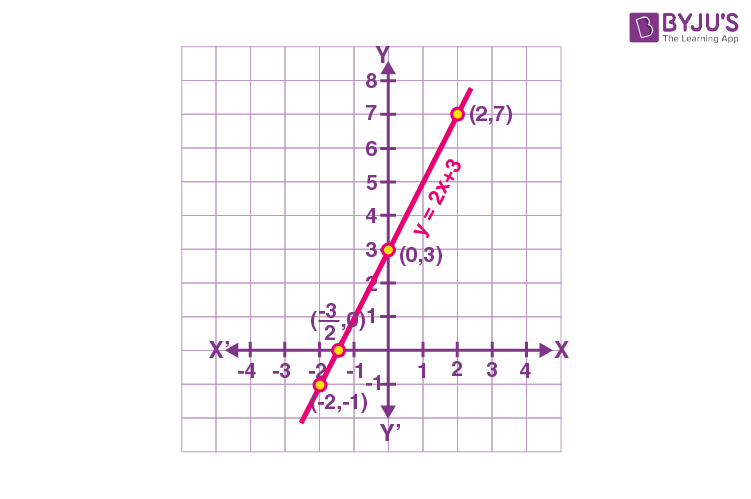From the graph, we can observe that graph y=2x+3 intersects the x-axis between x=-1 and x=-2

(i.e) The straight line intersects the x-axis at the point (-3/2, 0).

Therefore, -3/2 is the zero of the polynomial y=2x+3.

In general, we can say that a linear polynomial ax+b, where a≠0, has exactly one zero. The zero of the linear polynomial is the x-coordinate of the point where the graph of y=ax+b intersects at the x-axis.

Geometrical Meaning of Zeroes of Quadratic Polynomial:

We know that the standard form of a quadratic polynomial is ax2+bx+c, where a≠0. Now, let us understand the geometrical meaning of zeroes of quadratic polynomials with the help of an example.

Consider the quadratic equation, y= x2-3x-4

For the given quadratic equation, first find the coordinates (x, y), by taking a few values of x.

x

-2

-1

0

1

2

3

4

5

y=x2-3x-4

6

0

-4

-6

-6

-4

0

6

Hence, the coordinates formed are (-2, 6), (-1, 0), (0, -4), (1, -6), (2, -6), (3, -4), (4, 0) and (5, 6).

Now, graph the points as shown below: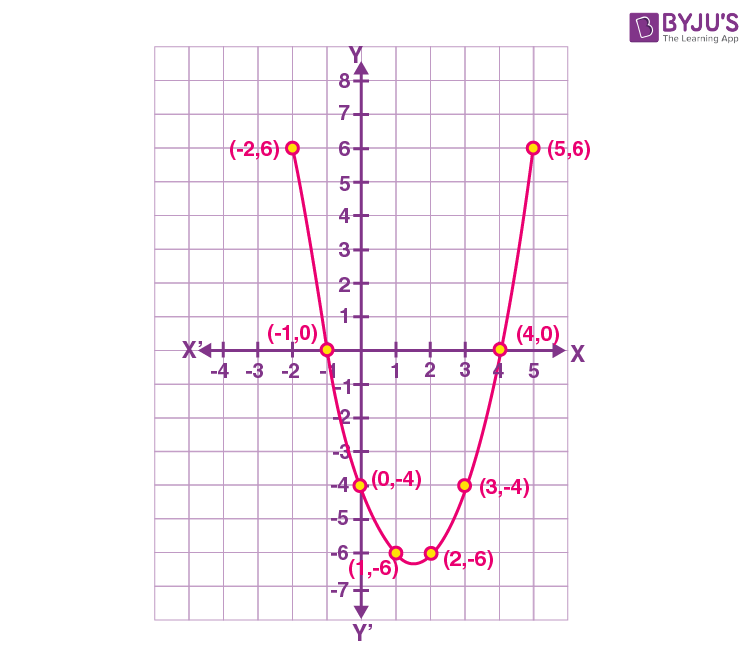Generally, the graph of the quadratic equation, y=ax2+bx+c, where a≠0, has two types of curves such as the parabolic curve open upwards or parabolic curve open downwards, depending on whether a>0 or a<0.

From the graph, we can observe that the two zeroes of the polynomial y = x2 – 3x – 4 are -1 and 4.

The zeroes -1 and 4 are the x-coordinates of the point in which the graph, y = x2 – 3x – 4 intersects at the x-axis.

Since the quadratic equation has at most two zeroes, there exist three different cases. They are:

Case 1: The graph cuts the x-axis at two distinct points, say A and A’.

In this case, the quadratic polynomial has two zeroes.

Example: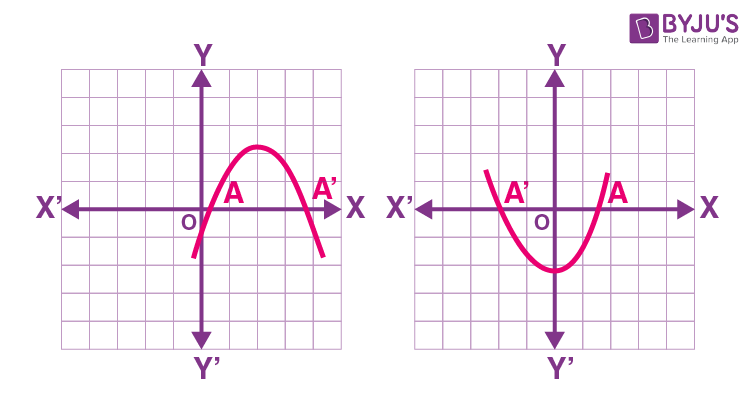Case 2: The graph cuts the X-axis at exactly one point, say A.

In this case, there exists only one zero.

Example: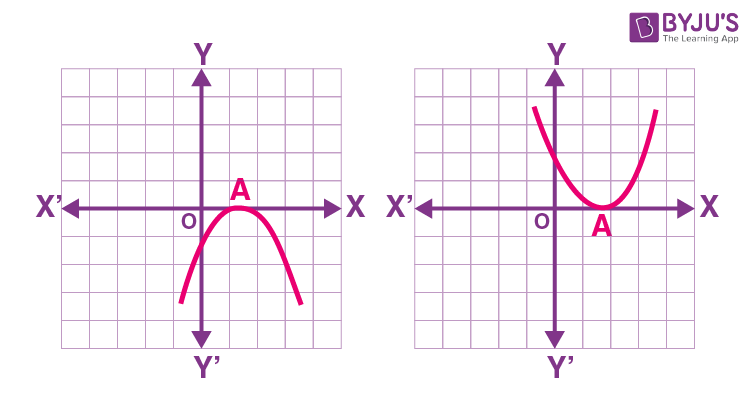Case 3: The graph does not cut X-axis at any point.

In this case, the curve for the given quadratic polynomial is completely above or below the x-axis. So, the quadratic polynomial has no zero in this case.

Example: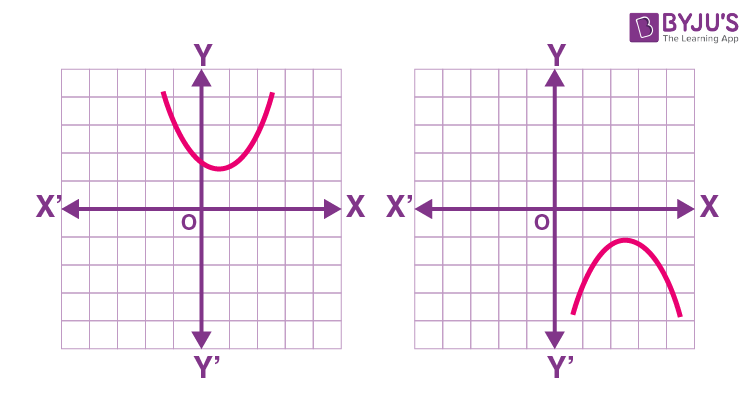### Practice Problems

Find the number of zeroes for the following graphs of polynomial equations: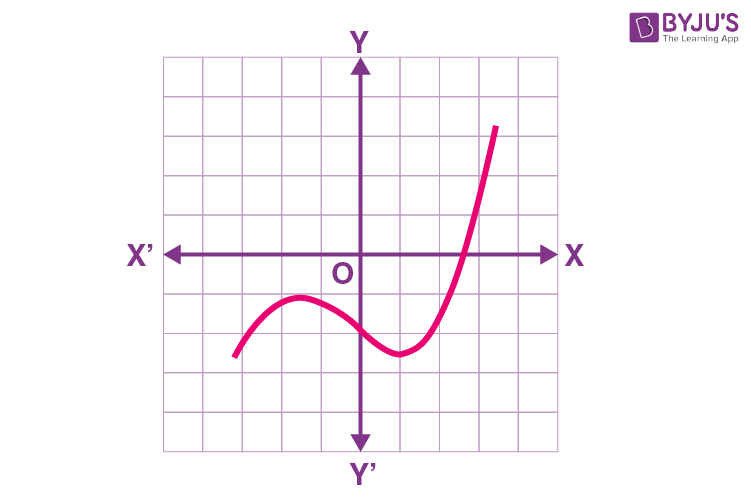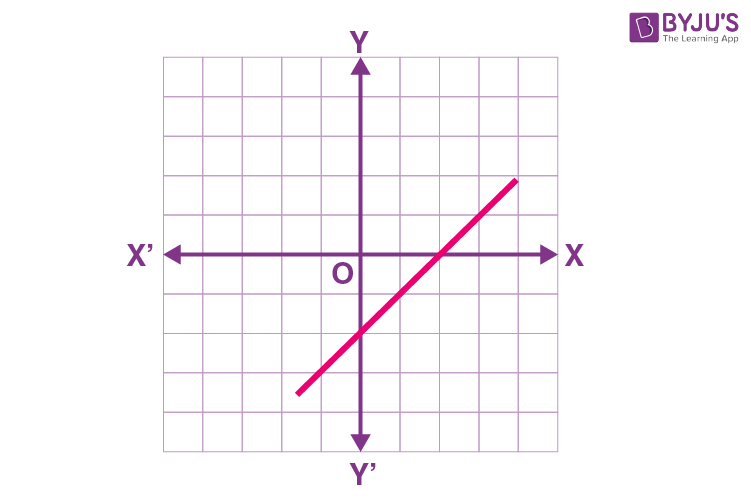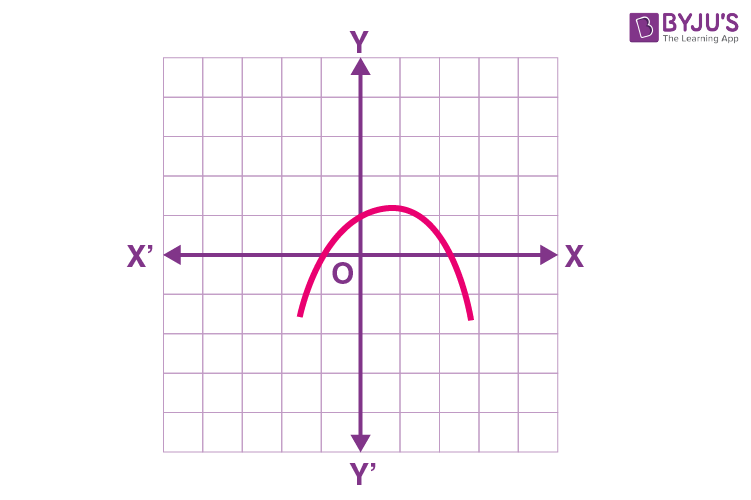Stay tuned with BYJU’S – The Learning App and download the app to learn all Maths-related concepts by exploring more videos.